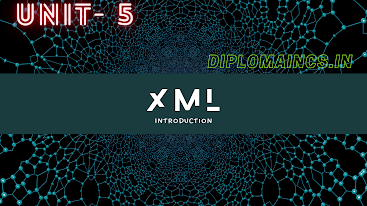## 12/29/22

### Digital Electronics and Microprocessor 2022 question

#### Digital Electronics and Microprocessor 2022

Sub Code: - 2018304

2022(Odd)

Time : 3Hrs

Semester  III/CSE

DE&M

Full Marks : 70

Pass marks : 28

Group A

Choose the most suitable answer from the following options: -   (1*20=20)

(i) Minimum number of NAND gate required to implement  full adder is

(a) 8

(b) 10

(c)  12

(d) 9

(ii) The most commonly used system for representing signed binary number is the:

(a) 2’s complement system

(b) 1’s complement system

(c) 10’s complement system

(d) Sign magnitude system

(iii) The logical expression for a NOR gate is:

(a) X=A’+B

(b) X=A+B’

(c) X=A+B

(d) X=(A+B)’

(iv) Binary coded decimal or BCD is also known as

(a) 2841

(b) 4821

(c) 4281

(d) 8421

(v) D- flip flop can be made from a J-K flip flop by making

(a) J=K

(b) J=K=1

(c) J=0, K=1

(d)J=K’

(vi) When two asynchronous active low inputs present and clear or applied to a J-K flip flop the output will be:

(a) Zero

(b) Undefined

(c) Previous state

(d) 1

(vii) In a positive edge triggered JK flip-flop, a low J and a low K produces:

(a) Low state

(b) Toggle state

(c) High state

(d)No change

(viii) The condition for making a J-K flip-flop made to toggle

(a) J=0, K=0

(b) J=1, K=0

(c) J=0, K=1,

(d) J=1, K=1

(ix) The main difference between a register and a counter is:

(a) A register has no specific sequence of states

(b) A counter has no specific sequence of states

(c) A register has capability to store one bit of information but counter has n-bit

(d) A register counts data

(x) Minimum number of NOR gates required to implement full adder is:

(a) 10

(b) 9

(c) 11

(d) 12

(xi) Minimum number of 4:1 mux required to implement 64:1 mux is:

(a) 23

(b) 21

(c) 24

(d) 20

(xii) If a 10-bit ring has an initial state 1101000000, What is the state after second clock pulse:

(a) 1101000000

(b) 0011010000

(c) 1100000000

(d) 0000000000

(xiii) A digital multiplexer is a combinational circuit that selects _________

(a) One digital information from several source and transmits the selected one

(b) Many digital information and convert them into one

(c) Many decimal inputs and transmits the selected information

(d) None of the above

(xiv)Convert binary into gray code 100101

(a)101101

(b) 001110

(c) 110111

(d) 111001

(xv) How many AND, OR and EX OR gates are required for the configuration of full adder?

(a)  1,2,2

(b) 2,1,2

(c)  3,1,2

(d) 4,0,1

(xvi) Convert binary number into decimal number (11011.1011)2 into decimal

(a) (27.6875)10

(b) (27.5874)10

(c) (26.9876)10

(d) (27.7893)10

(xvii) Master slave flip-flop is also referred to as

(a) Level triggered flip-flop

(b) Pulse triggered flip-flop

(c)  Edge triggered flip-flop

(d) Edge-Level triggered flip-flop

(xviii) How many inputs and outputs does a full adder have?

(a)  3,2

(b) 2,3

(c)  3,3

(d) 2,2

(xix) Which of the following addressing technique is not used in 8085 microprocessor?

(a) Register

(b) Immediate

(c) Register indirect

(d) Relative

(xx) Which of the following is ALE (bar) signal pin in 8086 microprocessor?

(a) Pin 27

(b) Pin 31

(c)  Pin 25

(d) Pin 26

Group:-"B"

Answer all Five Questions: -                         (5*4=20)

2.  Differentiate between multiplexer and demultiplexer.

OR

Explain the working o0f 4-bits register.

OR

Draw the logic diagram of full adder using NAND gates.

4. Design Ex-OR gates using NAND gates only.

OR

a.    Realize basic gates using NAND only.

b.    Convert (845)10 into its equivalent BCD and excess-3 code.

5.  Draw and explain the working of clocked R-S flip-flop with timing diagram.

OR

Draw and explain master slave JK flip-flop with truth table.

6.  Simplify the Boolean expression Y (A,B,C)=(0,4,5,6,7) using k-map and draw logic circuit after reduction of Boolean expression.

OR

Implement NAND gate using 2:1 MUX.

Group:- "C"

Answer all Five Questions: -                         (5*6=30)

7.  Simplify the following using k-map and implement using NAND-NAND gates only:

Y =(0,1,2,3,5,7,8,9,11)

OR

Simplify using k-map and implement using NOR-NOR gates only:

f(A,B,C,D) =(0,2,6,7,8,10,12,14,15)

8. Prove NOR gate and NAND gate as universal gate with suitable diagrams.

OR

Define modulus of counter and write down the number of flip-flop required to construct mod 7 counter.

9. Explain addressing modes of 8086 microprocessor with examples. Explain any three-arithmetic instruction of 8086.

OR

Draw and explain the functional block diagram of 8086 microprocessor. List different registers and state their functions in 8085 microprocessor.

10.

a.    Implement OR gate using 2:1 MUX using “n-1” select lines.

b.    Implement 16:1 MUX using 2:1 MUX.

OR

a.    Design half adder using NOR gates only.

c.     Design 8 to 3 encoder using 4 to 2 encoder.

11.  Write short notes on any two:

a.    Sequential circuits vs combinational circuits

b.    A/D converter

c.     Display Devices

OR

Explain different types of memories. Differentiate between static and dynamic memory.